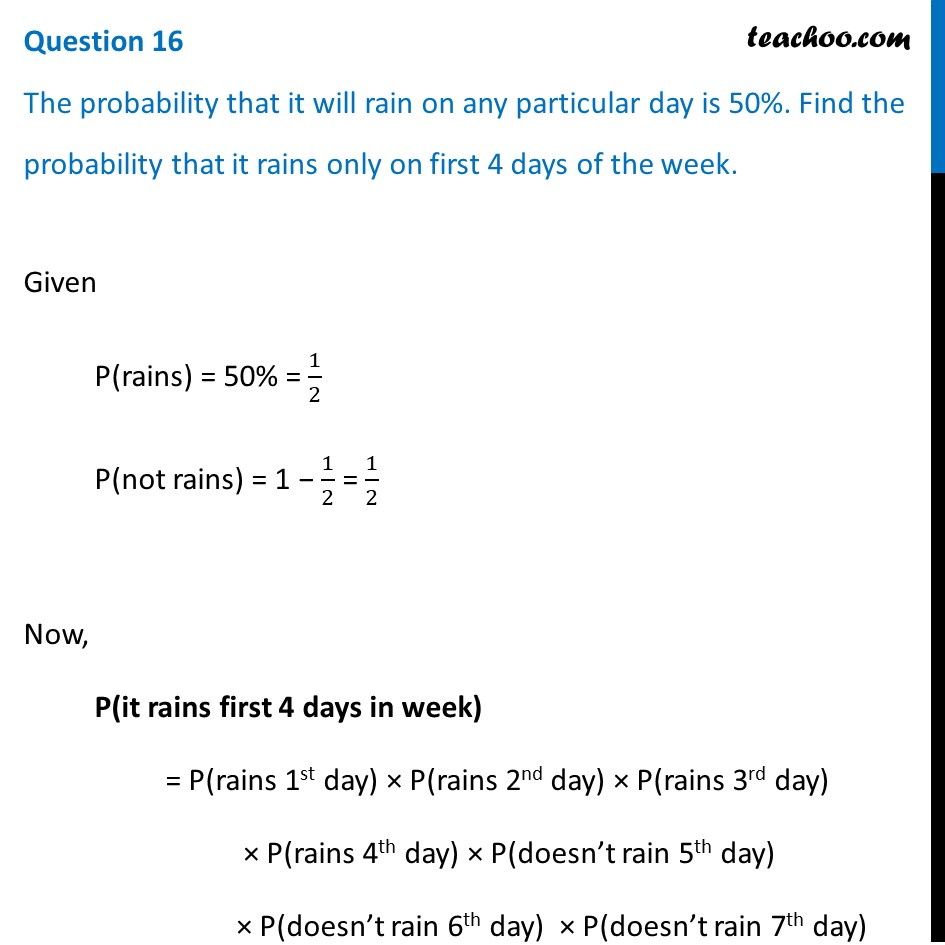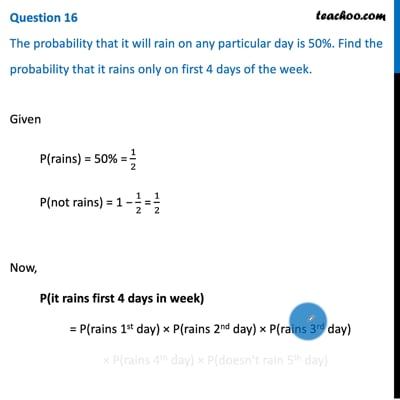CBSE Class 12 Sample Paper for 2021 Boards

Class 12
Solutions of Sample Papers and Past Year Papers - for Class 12 Boards

## The probability that it will rain on any particular day is 50%. Find the probability that it rains only on first 4 days of the week.This video is only available for Teachoo black users

Introducing your new favourite teacher - Teachoo Black, at only ₹83 per month

### Transcript

Question 16 The probability that it will rain on any particular day is 50%. Find the probability that it rains only on first 4 days of the week. Given P(rains) = 50% = 1/2 P(not rains) = 1 − 1/2 = 1/2 Now, P(it rains first 4 days in week) = P(rains 1st day) × P(rains 2nd day) × P(rains 3rd day) × P(rains 4th day) × P(doesn’t rain 5th day) × P(doesn’t rain 6th day) × P(doesn’t rain 7th day) = (𝟏/𝟐)^𝟒 × (𝟏/𝟐)^𝟑 = (1/2)^7 = 𝟏/𝟏𝟐𝟖